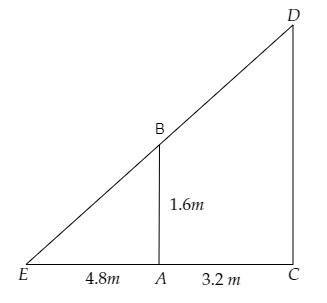# A $1.6 \mathrm{~m}$ tall girl stands at a distance of $3.2 \mathrm{~m}$ from a lamp-post and casts a shadow of $4.8$ m on the ground. Find the height of the lamp-post by using trigonometric ratios.

Given:

A $1.6 \mathrm{~m}$ tall girl stands at a distance of $3.2 \mathrm{~m}$ from a lamp-post and casts a shadow of $4.8$ m on the ground.

To do:

We have to find the height of the lamp-post by using trigonometric ratios.

Solution:Let $AB$ be the girl and $CD$ be the height of the lamp post.

Let $AE$ be the shadow of the girl.

From the figure,

$\mathrm{AC}=3.2 \mathrm{~m}, \mathrm{AE}=4.8 \mathrm{~m}$

Let the height of the lamp post be $\mathrm{DC}=h \mathrm{~m}$.

We know that,

$\tan\ BEA =\frac{\text { Opposite }}{\text { Adjacent }}$

$=\frac{\text { BA }}{EA}$

$=\frac{1.6}{4.8}$

$=\frac{1}{3}$.......(i)

Similarly,

$\tan\ DEC=\tan\ BEA=\frac{\text { Opposite }}{\text { Adjacent }}$

$=\frac{\text { DC }}{EC}$

$=\frac{h}{4.8+3.2}$

$=\frac{h}{8}$.........(ii)

From (i) and (ii), we get,

$\frac{1}{3}=\frac{h}{8}$

$\Rightarrow h=\frac{8}{3} \mathrm{~m}$

Therefore, the height of the lamp-post is $\frac{8}{3} \mathrm{~m}$.

Updated on: 10-Oct-2022

63 Views